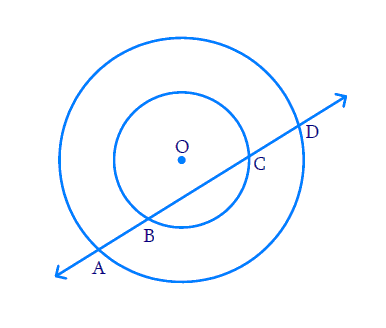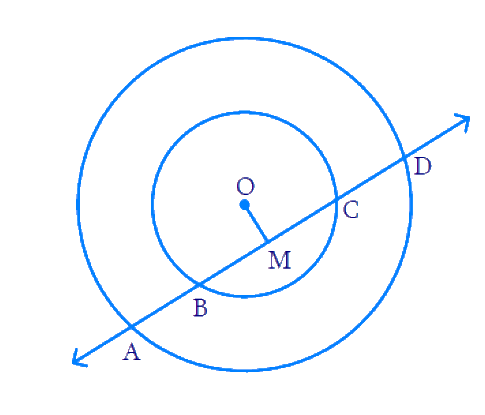# Ex.10.4 Q4 Circles Solution - NCERT Maths Class 9

Go back to  'Ex.10.4'

## Question

If a line intersects two concentric circles (circles with the same center) with centre $${O}$$ at $$A, B, C$$ and $$D$$, prove that $${AB = CD.}$$Video Solution
Circles
Ex 10.4 | Question 4

## Text Solution

What is known?

Two concentric circles with centre \begin{align} {O.} \end{align}

What is unknown?

Proof that $${AB = CD}$$

Reasoning:

Perpendicular drawn from the centre of the circle bisects the chord.

Steps:Draw a perpendicular from the centre of the circle $${OM}$$ to the line $${AD}$$.

We can see that $${BC}$$ is the chord of the smaller circle and $${AD}$$ is the chord of the bigger circle.

We know that perpendicular drawn from the centre of the circle bisects the chord.

\begin{align}∴ {BM}={MC} \ldots(1)\end{align}

And, $$AM = MD ... (2)$$

Subtracting ($$2$$) from ($$1$$), we obtain

\begin{align}{AM - BM }= {MD} - {MC}\end{align}

\begin{align}∴ {AB = CD}\end{align}

Video Solution
Circles
Ex 10.4 | Question 4

Learn from the best math teachers and top your exams

• Live one on one classroom and doubt clearing
• Practice worksheets in and after class for conceptual clarity
• Personalized curriculum to keep up with school